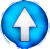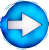Factorizing the biggest common factor using common factor methodGo ! $2a+2b = 2(a + b)$ (sum of 2 terms -> productof 2 factors ! $2a+ab = a(2 + b)$ $a^2+a = a^2+1a = a(a+1)$ $a+ab+2ac$ = $x^2-x$ = $a(x-1)+b(x-1)$ = $a(x-1)+(1-x)b$ = $2a^2b-4ab$ =

>